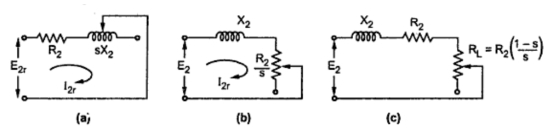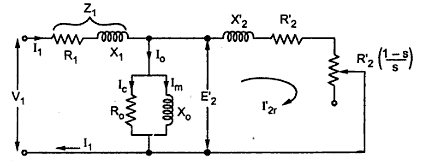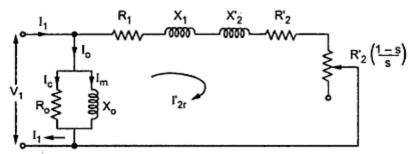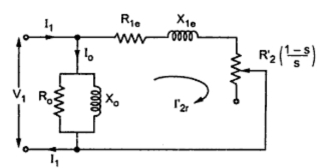Equivalent Circuit of Induction Motor : Part 1

We have already seen that the induction motor can be treated as generalized transformer. Transformer works on the principle of electromagnetic induction. The induction motor also works on the same principle. The energy transfer from stator to rotor of the induction motor takes place entirely with the help of a flux mutually linking the two. Thus stator acts as a primary while the rotor acts as a rotating secondary when induction motor is treated as a tranformer.
If                            E1 = Induced voltage in stator per phase
E2 = Rotor induced e.m.f. per phase on standstill
k = Rotor turns / Stator turns
then                          k = E2/ E1
Thus if  V1 is the supply voltage per phase to stator, it produces the flux which links with both stator and rotor. Due to self induction E1, is the induced e.m.f. in stator per phase while  E2 is the induced e.m.f. in rotor due to mutual induction, at standstill. In running condition the induced e.m.f. in rotor becomes E2r which is s E2.
Now                   E2r  = Rotor induced e.m.f. in running condition per phase
R= Rotor resistance per phase
X2r  = Rotor reactance per phase in running condition
R= Stator resistance per phase
X= Stator reactance per phase
So induction motor can be represented as a transformer as shown in the Fig. 1.Fig. 1 Induction motor as a transformer
When induction motor is on no load, it draws a current from the supply to produce the flux in air gap and to supply iron losses.
1. I= Active component which supplies no load losses
2. I= Magnetizing component which sets up flux in core and air gap
These two currents give us the elements of an exciting branch as,
R= Representing no load losses = V1 /I
and              X= Representing flux set up = V1/Im
Thus,            Īo = Īc + Īm
The equivalent circuit of induction motor thus can be represented as shown in the Fig. 2.Fig. 2  Basic equivalent circuit
The stator and rotor sides are shown separated by an air gap.
I2r   = Rotor current in running condition
= E2r /Z2r   = (s E2)/√(R22 +(s X2)2)
It is important to note that as load on the motor changes, the motor speed changes. Thus slip changes. As slip changes the reactance X2r  changes. Hence X2r  = sX2 is shown variable.
Representing of rotor impedance :
It is shown that,         I2r  = (sE2)/√(R22 +(s X2)2) = E2 /√((R2/s)2 + X22)
So it can be assumed that equivalent rotor circuit in the running condition has fixed reactance X2, fixed voltage E2 but a variable resistance R2/s, as indicated in the above equation.
So the variable rotor resistance R2/s has two parts.
1. Rotor resistance R2 itself which represents copper loss.
2. R2(1 - s)/s which represents load resistance RL. So it is electrical equivalent of mechanical load on the motor.
Key Point : Thus the mechanical load on the motor is represented by the pure resistance of value R2(1 -s)/s.
So rotor equivalent circuit can be shown as,Fig.  3   Rotor equivalent circuit
Now let us obtain equivalent circuit referred to stator side.
Equivalent circuit referred to stator :
Transfer all the rotor parameters to stator,
k = E2/E1 = Transformation ratio
E2' = E2/ k
The rotor current has its reflected component on the stator side which is I2r'.
I2r' = k I2r = (k s E2 )/√(R22 +(s X2)2)
X2' = X2/K2 = Reflected rotor reactance
R2' = R2/K2 = Reflected rotor resistance
RL' = RL/K2 = (R2/K2)(1-s / s)
= R2' (1-s / s)
Thus RL' is reflected mechanical load on stator.
So equivalent circuit referred to stator can be shown as in the Fig. 4Fig. 4   Equivalent circuit referred to stator
.
The resistance R2' (1 -s)/ s = RL' is fictitious resistance representing the mechanical load on the motor.
1.1 Approximate Equivalent Circuit
Similar to the transformer the equivalent circuit can be modified by shifting the exciting current (Ro and Xo) purely across the supply, to the left of R1 and X1. Due to this, we are neglecting the drop across  R1 and X1 due to Io, which is very small. Hence the circuit is called approximate equivalent circuit. The circuit is shown in the Fig.5.Fig. 5  Approximate equivalent circuit
Now the resistance R1 and R2' while reactance X1 and X2' can be combined. So we get,
R1e = Equivalent resistance referred to stator = R1 + R2'
X1e = Equivalent reactance referred to stator = X1 + X2'
R1e = R1 + (R2/K2)
and              X1e = X1 + (X2/K2)
While           Ī1 =  Īo + Ī2r'               .........phasor diagram
and               Īo = Īc + Īm
Thus the equivalent circuit can be shown in the Fig.6.Fig.  6

Power Equations from Equivalent Circuit
With reference to approximate equivalent circuit shown in the Fig. 6, we can write various power equations as,
Pin = input power = 3 V1 I1 cos Φ
where    V1 = Stator voltage per phase
I1 = Current drawn by stator per phase
cos Φ = Power factor of stator
Stator core loss  =  Im2 Ro
Stator copper loss = 3 I12 Ro
where             R1 = Stator resistance per phase
P = Rotor input = (3 I2r'2 R2')/s
P = Rotor copper loss = 3 I2r'2 R2'
Thus               P = s P
P = Gross mechanical power developed

T = Torque developed

where             N = Speed of motor
But                 N = N (1-s) =, so substituting in above

and                 I2r' = V1 /(( R1e + RL') + j X1e )
where             RL' = R2' (1-s)/s
I2r' = V1/√(( R1e + RL')2 + X1e2 )
Key Point : Remember that in all the above formula all the values per phase values.

See part 2